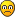Hello Everyone,

I'm trying to calculate the acceleration duration for a certain heave displacement in order to generation 0.5G (4.905 m/s²) of accelearation.

I'm using CKAS U5 3DOF Motion Syteme http://www.ckas.com.au/3dof_systems_64.html to double check my calculations and cannot seem to be able to reproduce their results which are:
displacement =0.140 m
velocity=0.280 m/s
acceleration=4.905 m/s²

I know that:
The Time t taken for an object to fall distance d is t=SQRT(2d/g)
Instantaneous velocity vi of a falling object after elapsed time t is vi = gt
The Instantaneous velocity vi of a falling object that has traveled distance d is vi = SQRT(2gd)

My guest is that we need one half of the displacement to accelerate to speed and the other half in reserve to stop moving.

This would mean that (0.14/2)=0.07 would be the actual length available for the acceleration meaning that:
t = SQRT(2x0.070/4.905) = 0.169 s

Trying to get back to the CKAS velocity of 0.280 m/s I'm getting
vi = gt = 4.905x0.169 = 0.829 m/s
vi = SQRT(2gd) = SQRT(2x4.905x0.07) = 0.829 m/s

0.829 m/s is far for been equal to 0.280 m/s

This is so frustrating.I must be missing something so basic.Could someone tell me what I'm doing wrong.
Marc T.marc

Posts: 3
Images: 2
Joined: Thu 5. Feb 2015, 00:24
Has thanked: 0 time
Been thanked: 0 time